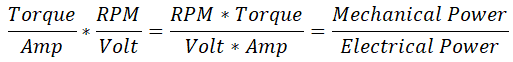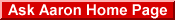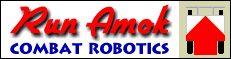# Converting basic electric motor specs to motor constants.from Team Run Amok

Converting Motor Specs

Finding Kv, Kt and Stall Amps

Q: I'd like to see if a pair of 36 Volt 1000 Watt 3000 RPM Electric Scooter Motors will work for my 'bot, but the motors have no specs. Is there any way I can use the Tentacle Torque Calculator to see what the performance of my 'bot would be with these motors?

A: You have more motor information than you may think. You can get the Voltage Constant (Kv) from the voltage and speed:

Kv = RPM ÷ volts

Kv = 3000 ÷ 36

Kv = 83.3

Once you have the Voltage Constant (Kv) you can calculate the Torque Constant (Kt) by taking advantage of a counterintuitive relationship between Kv and Kt. For inch-ounce torque units, Kt × Kv = 1352. A little algebra gives:

Kt = 1352 ÷ Kv

Kt = 1352 ÷ 83.3

Kt = 16.2

Brushed motors typically report their output power in 'peak watts', which can provide a fair estimate of current at stall:
Stall Amps = 4 × Peak Watts ÷ voltage

Stall Amps = 4 × 1000 ÷ 36

Stall Amps = 111

Note that hobby brushless motors generally report their output power as 'continuous watts' -- the power the motor can sustain without thermal overload. A 'continuous watts' power rating cannot be used for this stall amps formula.

Now that you know the math, let me save you some time. I've written a JavaScript 'Motor Spec Converter' to quickly convert motor RPM, voltage, and output watts into the inputs needed for the Tentacle calculator. Fill in the blue input cells with your values and click the 'Calculate' button.

 Motor Spec Converter Speed: RPM Voltage: volts Output: watts Kv: RPM / volt Kt: oz-in / amp Stall: amps

Q: Wait... Wait... How is it that Kt × Kv always equals 1352? That makes no sense at all. I mean, different motors should have completely different speed and torque constants that have no relationship to each other! What gives???

A: I did warn that it was counterintuitive. It helps to look at the two constants in fraction form and then examine the units their product represents:

• Kt = torque/amp
• Kv = RPM/volt
Their product then becomes:So, the product of Kt and Kv becomes the ratio of your chosen units of mechanical power to your units of electrical power -- which is constant.
Q: Hold on... then why are stall amps = 4 × Peak Watts ÷ voltage?

A: Not gonna take that on faith, huh? OK, take a look at the chart below. It shows the relationship between RPM, torque, Amps, and Output Power for a permanent magnet direct current (PMDC) motor.As noted in the answer to the above question, mechanical output power is the product of torque and RPM. Peak output power occurs with the motor loaded to 50% of maximum torque, which happens at 50% of the motor's maximum RPM. At that load the motor is drawing 50% of its stall current. So:
(Stall Amps ÷ 2) × Voltage = Power Consumed at Peak Output Watts
Now take note of the green line on the chart labeled 'Efficiency' that shows the relationship between electrical power input and mechanical power output. At peak output power the motor efficiency is 50% -- mechanical power output is half the electrical power consumed. Adjusting the above equation for efficiency gives:
(Stall Amps ÷ 4) × Voltage = Peak Output Watts
Multipy both sides of the equation by 4 and divide both sides by volts and you get:
Stall Amps = 4 × Peak Watts ÷ Voltage

Want more motor math? Team RoboForge Motor Math

 To see the most recent questions or to ask a new question, go to the